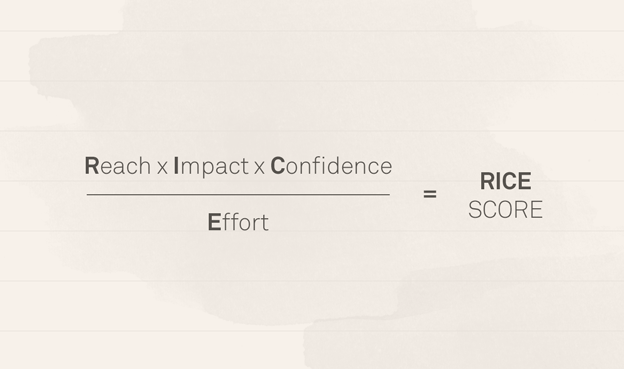Decision Making Frameworks
Share
Explore
Hello 👋

#RICE (Intercom)RICE (Intercom)

Source: (Intercom)

RICE is an acronym for the four factors we use to evaluate each project idea:
Reach: how many people will this impact? (Estimate within a defined time period.)
Impact: how much will this impact each person? (Massive = 3x, High = 2x, Medium = 1x, Low = 0.5x, Minimal = 0.25x.)
Confidence: how confident are you in your estimates? (High = 100%, Medium = 80%, Low = 50%.)
Effort: how many “person-months” will this take? (Use whole numbers and minimum of half a month – don’t get into the weeds of estimation.)